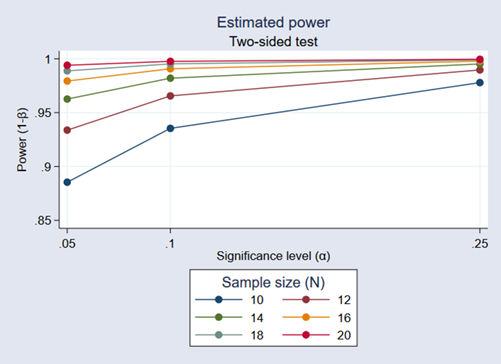»  Home »  Products »  Features »  Add your own power and sample-size methods

The power command provides many built-in methods, but sometimes, you may want to compute sample size or power yourself. For example, you may need to do this by simulation, or you may want to use a method that is not available in any software package. power makes it easy for you to add your own method. All you need to do is to write a program that computes sample size, power, or effect size, and the power command will do the rest for you. It will deal with the support of multiple values in options and with automatic generation of graphs and tables of results.

Suppose you want to add the method called mymethod to the power command. Just follow these three steps:

1. Create a program that computes sample size, power, or effect size and follows power's naming convention: power_cmd_mymethod.
2. Store results following power's simple naming conventions for results. For example, store the value of power in r(power), the value of sample size in r(N), and so on.
3. Place your program power_cmd_mymethod where Stata can find it.

To show how easy this all is, we'll write a program to compute power for a one-sample z test given sample size, standardized difference, and significance level. For simplicity, we assume a two-sided test.

We will call our new method myztest.


program power_cmd_myztest, rclass
version 16.0
//  parse options
syntax , n(integer)         sample size
STDDiff(real)      standardized diff.
Alpha(string)      significance level

//  compute power
tempname power
scalar power' = normal(stddiff'*sqrt(n') -
invnormal(1-alpha'/2))

// return results
return scalar power   = power'
return scalar N       = n'
return scalar alpha   = alpha'
return scalar stddiff = stddiff'
end



The computation in this program takes only one line, but it could be as complicated as we like. It could even involve simulation to compute the power.

With our program in hand, we can type

. power myztest, n(20) stddiff(1) alpha(.05)


power will find our program, supply it with the n(20), stddiff(1), and alpha(.05) options, and use its returned results to produce

. power myztest, n(20) stddiff(1) alpha(.05)

Estimated power
Two-sided test

alpha   power       N

.05    .994      20



That wasn't too impressive. Our program did all the work.

But what if we supplied power with a list of sample sizes?

. power myztest, n(10 15 20 25) stddiff(1)

Estimated power
Two-sided test

alpha   power       N

.05   .8854      10

.05   .9721      15

.05    .994      20

.05   .9988      25



power has taken our list of sample sizes and computed powers for all of them—even though our program could compute only a single power!

Moreover, we can use power's standard table() option to control exactly how that table looks. power also has hooks that let our program determine how the columns are labeled and how the table appears.

We can supply both sample sizes and significance levels and request a graph instead of a table:

. power myztest, n(10(1)20) alpha(.05 .10 .25) stddiff(1) graphWe can even request that the graph show α on the x axis with separate plots for each sample size.

. power myztest, n(10(2)20) alpha(.05 .10 .25) stddiff(1) graph(xdim(alpha))Now all this may just make it worth writing more complicated programs to compute power for more complicated tests and comparisons.